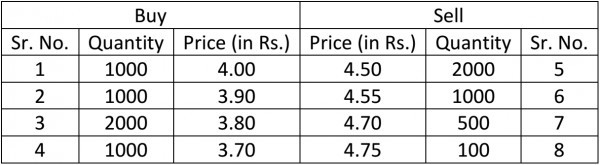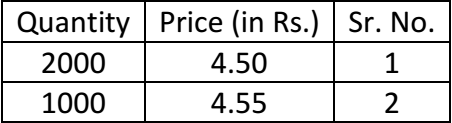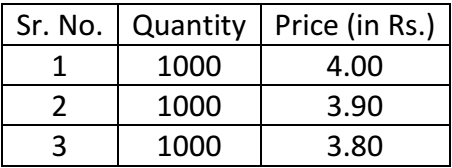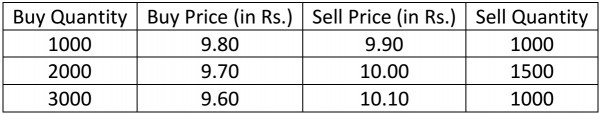# What is impact cost?

Hello,

When large orders are executed without noticable movement in stock prices, one can say the stock market is liquid.

Lets consider the snap quote of a stock at a point in time:The snap quote here consists of 4 best buy and 4 best sell orders.

Buyers can execute their orders at best seller prices and sellers can execute their orders at best buyer prices.
Here, a buy market order for 100 shares will get executed at 4.50 and a sell market order will get executed at 4.

Now, if a person buys 100 shares and immediately sells it, he will take a loss equal to the bid-ask spread into the quantity he holds.

In this case, his loss would be 0.05x100 = Rs.50.

This is regarded as the transaction cost which the market charges for the priviledge of trading.

Now lets increase the quantity and make it 3000 shares to transact.

If a person buys 3000 shares and immediately sells it,

The buy order will hit the following sell orders:And the sell order will hit the following buy orders:The transaction cost increases with increase in quantity. The bid-ask spread reflects transaction costs only for smaller quantities.

Lets consider what we now call Impact cost:

Lets define the ideal trading price as the average of the best bid and ask prices.
In an infinitely liquid market, both buy and sell orders should execute at this ideal price.

In the following example:To buy 1500 shares, Ideal Price = (9.8+9.9)/2 = Rs.9.85

Actual buy price = [(1000*9.9)+(500*10)]/1500 = Rs.9.93.

This difference is the Impact Cost.

Impact cost for buying 1500 shares = 9.93-9.85 = 0.08.

Impact cost in % = [(9.93-9.85)/9.85]*100 = 0.84%

Cheers!

1 Like

I understand the mathematics behind impact cost.

My doubt is how to use impact cost to identify liquid stocks.

What values indicate high liquidity? Like what range (0-1),(0-0.02) etc?